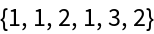Function Repository Resource:

# HyperellipticODE

Get a physicist's constructive proof of Fuchs's theorem on hyperelliptic curves

 ResourceFunction["HyperellipticODE"][V,{p,q, α}] returns the ODE constraining integral periods T(α) associated with a Hamiltonian constraint of the form α=2H(p,q)=p2+2V(q), and V(q) a non-linear q-polynomial. ResourceFunction["HyperellipticODE"][V,{p,q,α},certificate] also returns a checked and checkable Association containing proof data, when certificate is set to True.

## Details

The acronym ODE stands for "Ordinary Differential Equation".
Specification of variables {p, q, α} is optional if the potential is written in terms of the formal variable q.
For our purposes, a rational, elliptic or hyperelliptic level curve may be written in set-builder notation as:

𝒞(α)={(p,q)∈2:α=2H(p,q)=p2+2V(q):V(q)=n=1Nvnqn :vn∈ℝ }

Also, at least one vn with n>1 must not equal to zero.
When viewed in four dimensional space, such a level curve is really a level surface with measurable genus.
Whether the curve is rational, elliptic, or hyperelliptic depends on the degree cutoff N as well as particular values of the vn.
ResourceFunction["HyperellipticODE"] does not distinguish between harmonic, elliptic, or hyperelliptic curves.
ResourceFunction["HyperellipticODE"] cannot accept linear potentials as input because linear potentials do not admit periodic solutions.
Time t is defined in terms of the tangents Dt{p, q}={-qH,pH}.
The invariant time differential may then be written most directly as dt=dq/p.
Subsequent α-derivatives of the integrand dt are written as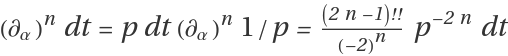.
The primary task of ResourceFunction["HyperellipticODE"] is to compute a set of coefficients cn(α) and a certificate Ξ(q, p) such that: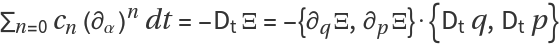When this condition is satisfied, the exact differential can be integrated to zero around a contour, which implies: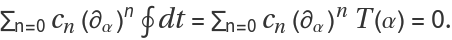This is the form of the minimal output, an ordinary differential equation constraining period functions T(α).
The proof data described above can be put into an Association:
 Potential V Hamiltonian α = 2H Coordinates {p, q,α} Tangents {-∂qH,∂pH} Time Forms (∂α)ndt,n=0, 1, … ODE Coefficients cn,n=0, 1, … Certificate Function Ξ Truth Value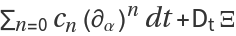The last item with Key "Truth Value" should auto-evaluate to 0 for valid data.
For more details refer to "An Update on the Computational Theory of Hamiltonian Period Functions", especially Chapter 3.

## Examples

### Basic Examples (5)

Find the period ODE coefficients of an elliptic curve:

 In:=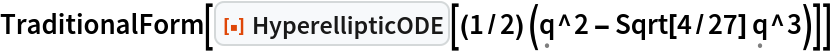Out=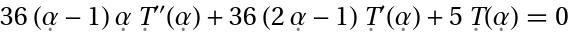Solve the ODE to find the real-valued hypergeometric period function:

 In:=Out=Display the proof data in a table:

 In:=Out=Write and check an unusual proof that the harmonic oscillator has a constant period:

 In:=Out=Continue on to a random hyperelliptic curve with terms up to q6:

 In:=Out=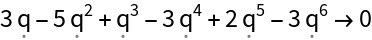### Scope (2)

Potentials can be specified with undetermined coefficients:

 In:=Out=In:=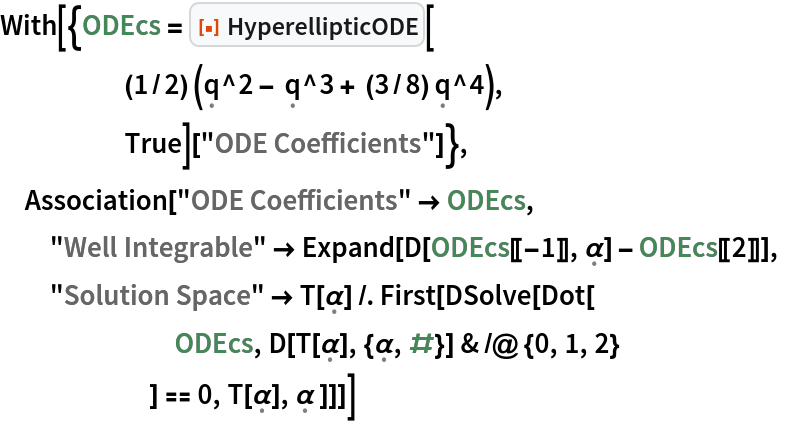Out=### Properties and Relations (1)

Proof data is dynamically checkable in a short time:

 In:=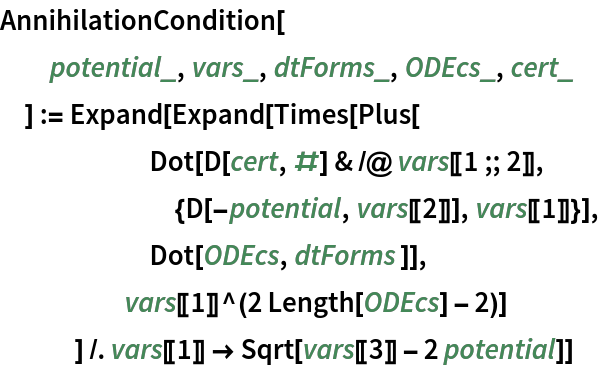In:=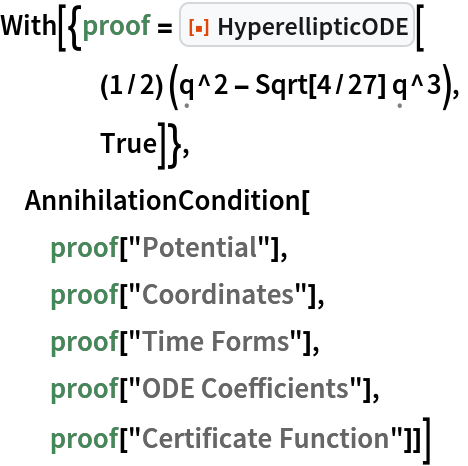Out=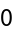### Possible Issues (1)

Inputting a linear potential function returns a failure:

 In:=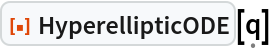Out=### Neat Examples (1)

Discover an apparent-genus-degree relation:

 In:=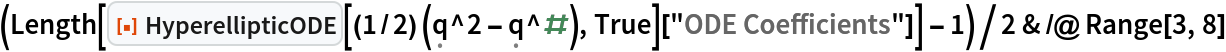Out=